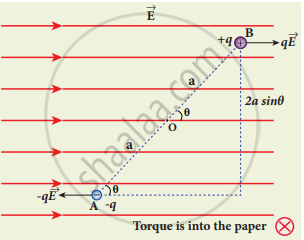Tamil Nadu Board of Secondary EducationHSC Science Class 12th

# Derive an expression for the torque experienced by a dipole due to a uniform electric field. - Physics

Derive an expression for the torque experienced by a dipole due to a uniform electric field.

#### Solution

1. Consider an electric dipole of dipole moment vec"p" placed in a uniform electric field. The charge +q will experience a force q vec"E" in the direction of the field and charge - q vec"E" will experience a force -qE in a direction opposite to the field. Since the external field vec"E" is uniform, the total force acting on the dipole is zero.
2. These two forces acting at different points will constitute a couple and the dipole experience a torque. This torque tends to rotate the dipole.
3. The total torque on the dipole about the point 0, is given by
vectau = vec"0A" xx (- "q"vec"E") + vec"0B" xx "q"vec"E"
Torque on dipole.
4. Total torque is perpendicular to the plane of the paper and is directed into it. The magnitude of the total torque
vectau = |vec"0A"| (- "q"vec"E") |sin theta + |vec"0B"| "q" vec"E"| sin thetaTorque on dipole
τ = qE.2a sin θ
5. Where θ is the angle made by vec"p" with vec"E". Since p = 2aq, the torque is written in terms of the vector product as
vec tau = vec"p" xx vec"E"
6. The magnitude of the torque is τ = pE sin θ and is maximum when θ = 90o.
This torque tends to rotate the dipole and align it with the electric field vec"E". once vec"p" is aligned with, the total torque on the dipole becomes zero.
7. If the electric field is nonuniform, then the force experienced by +q is different from that experienced by -q. In addition to the torque, there will be a net force acting on the dipole.
Concept: Electric Dipole and Its Properties
Is there an error in this question or solution?

#### APPEARS IN

Tamil Nadu Board Samacheer Kalvi Class 12th Physics Volume 1 and 2 Answers Guide
Chapter 1 Electrostatics
Evaluation | Q III. 5. | Page 74
Share